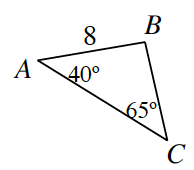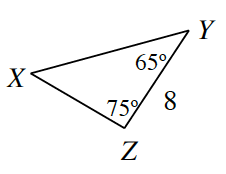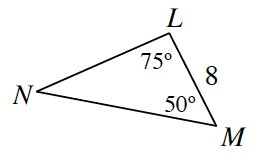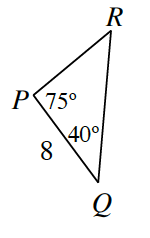### Home > GC > Chapter 6 > Lesson 6.2.1 > Problem6-46

6-46.

Decide if each triangle below is congruent to $ΔABC$ at right, similar but not congruent to $ΔABC$, or neither. Justify each answer.1.Find the missing angles using the Triangle Angle Sum Theorem.

Similar because of $\text{AA}\sim$, but not congruent.

1.Not similar and not congruent because there are no corresponding congruent angles between the triangles.

1.